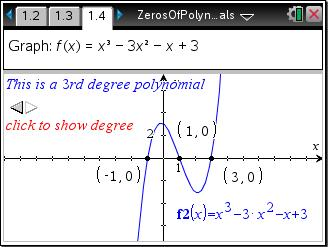Activities

••• Subject Area

• Math: Algebra II: Polynomials

• Author9-12

15 Minutes

• Device
•TI-Nspire™ CX/CX II
•TI-Nspire™ CX CAS/CX II CAS
• TI-Nspire™
• TI-Nspire™ CAS
• Software

TI-Nspire™
TI-Nspire™ CAS

3.2

• Report an Issue

Zeros of Polynomials

Activity Overview

Students graph polynomials to determine the value and number of zeros for a given polynomial.

Key Steps

•Students will graph different degree polynomial equations to determine the value and number of zeros for a given equation. They will use the Intersection Points tool and the Coordinates and Equations tool to find and display the zeros.

•They are to make a conjecture about the number of zeros and the degree of the polynomial and answer several questions related to the conjecture.